# Hough Circle Transform¶

## Goal¶

In this tutorial you will learn how to:

• Use the OpenCV function HoughCircles to detect circles in an image.

## Theory¶

### Hough Circle Transform¶

• The Hough Circle Transform works in a roughly analogous way to the Hough Line Transform explained in the previous tutorial.

• In the line detection case, a line was defined by two parameters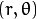. In the circle case, we need three parameters to define a circle: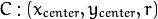where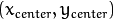define the center position (green point) and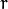is the radius, which allows us to completely define a circle, as it can be seen below: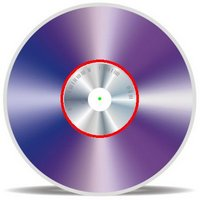• For sake of efficiency, OpenCV implements a detection method slightly trickier than the standard Hough Transform: The Hough gradient method, which is made up of two main stages. The first stage involves edge detection and finding the possible circle centers and the second stage finds the best radius for each candidate center. For more details, please check the book Learning OpenCV or your favorite Computer Vision bibliography

## Code¶

1. What does this program do?
• Loads an image and blur it to reduce the noise
• Applies the Hough Circle Transform to the blurred image .
• Display the detected circle in a window.
2. The sample code that we will explain can be downloaded from here. A slightly fancier version (which shows trackbars for changing the threshold values) can be found here.
#include "opencv2/highgui.hpp"
#include "opencv2/imgproc.hpp"
#include <iostream>
#include <stdio.h>

using namespace cv;

/** @function main */
int main(int argc, char** argv)
{
Mat src, src_gray;

src = imread( argv, 1 );

if( !src.data )
{ return -1; }

/// Convert it to gray
cvtColor( src, src_gray, CV_BGR2GRAY );

/// Reduce the noise so we avoid false circle detection
GaussianBlur( src_gray, src_gray, Size(9, 9), 2, 2 );

vector<Vec3f> circles;

/// Apply the Hough Transform to find the circles
HoughCircles( src_gray, circles, CV_HOUGH_GRADIENT, 1, src_gray.rows/8, 200, 100, 0, 0 );

/// Draw the circles detected
for( size_t i = 0; i < circles.size(); i++ )
{
Point center(cvRound(circles[i]), cvRound(circles[i]));
// circle center
circle( src, center, 3, Scalar(0,255,0), -1, 8, 0 );
// circle outline
circle( src, center, radius, Scalar(0,0,255), 3, 8, 0 );
}

namedWindow( "Hough Circle Transform Demo", CV_WINDOW_AUTOSIZE );
imshow( "Hough Circle Transform Demo", src );

waitKey(0);
return 0;
}


## Explanation¶

src = imread( argv, 1 );

if( !src.data )
{ return -1; }

2. Convert it to grayscale:

cvtColor( src, src_gray, CV_BGR2GRAY );

3. Apply a Gaussian blur to reduce noise and avoid false circle detection:

GaussianBlur( src_gray, src_gray, Size(9, 9), 2, 2 );

4. Proceed to apply Hough Circle Transform:

vector<Vec3f> circles;

HoughCircles( src_gray, circles, CV_HOUGH_GRADIENT, 1, src_gray.rows/8, 200, 100, 0, 0 );


with the arguments:

• src_gray: Input image (grayscale).
• circles: A vector that stores sets of 3 values: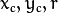for each detected circle.
• CV_HOUGH_GRADIENT: Define the detection method. Currently this is the only one available in OpenCV.
• dp = 1: The inverse ratio of resolution.
• min_dist = src_gray.rows/8: Minimum distance between detected centers.
• param_1 = 200: Upper threshold for the internal Canny edge detector.
• param_2 = 100*: Threshold for center detection.
• min_radius = 0: Minimum radio to be detected. If unknown, put zero as default.
• max_radius = 0: Maximum radius to be detected. If unknown, put zero as default.
5. Draw the detected circles:

for( size_t i = 0; i < circles.size(); i++ )
{
Point center(cvRound(circles[i]), cvRound(circles[i]));
// circle center
circle( src, center, 3, Scalar(0,255,0), -1, 8, 0 );
// circle outline
circle( src, center, radius, Scalar(0,0,255), 3, 8, 0 );
}


You can see that we will draw the circle(s) on red and the center(s) with a small green dot

6. Display the detected circle(s):

namedWindow( "Hough Circle Transform Demo", CV_WINDOW_AUTOSIZE );
imshow( "Hough Circle Transform Demo", src );

7. Wait for the user to exit the program

waitKey(0);


## Result¶

The result of running the code above with a test image is shown below: